What does it do?

Returns a random integer number between the numbers you specify.

Formula breakdown:

=RANDBETWEEN(bottom number, top number)

What it means:

=RANDBETWEEN(10, 10000)

I use the RANDBETWEEN function all the time whenever I need to create a sample data set.

The cool thing about the RANDBETWEEN function is that if you don’t like the numbers that it has given you, you can press F9 in a cell and it will give you new numbers.  Try it out by downloading the sample workbook!We want to enter some random numbers from 10 to 10,000.

STEP 1: We need to enter the RANDBETWEEN function in a blank cell:

## =RANDBETWEEN(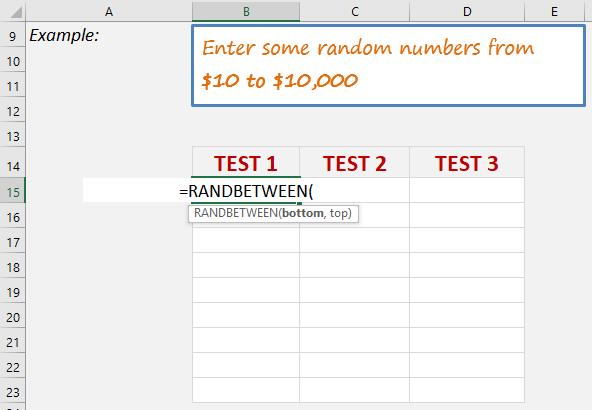STEP 2: The RANDBETWEEN arguments:

## Bottom_number

For our example it’s 10

## =RANDBETWEEN(10,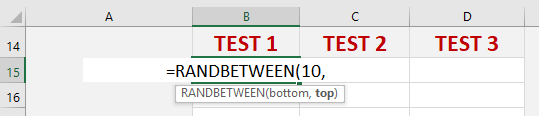## Top_number

For our example it’s 10,000

## =RANDBETWEEN(10, 10000)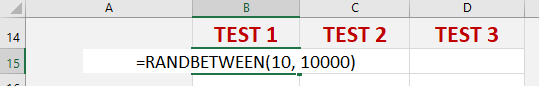Apply the same formula to the rest of the cells by dragging the lower right corner downwards. Then drag it to the right to populate all the cells.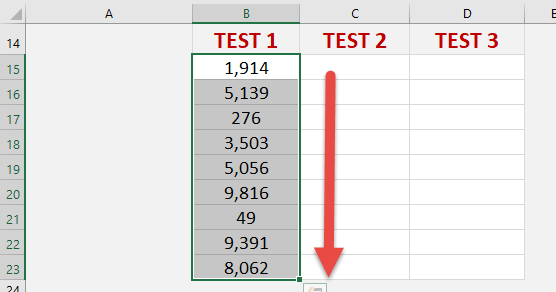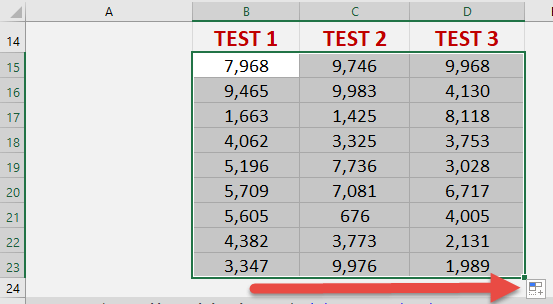You now have all of the random numbers!

How to Use the Randbetween Formula in Excel